#Function Repository Resource:

# FormatAsResourceFunction

Format a symbol as a ResourceFunction in outputs

Contributed by: Richard Hennigan (Wolfram Research)
 ResourceFunction["FormatAsResourceFunction"][symbol] formats symbol as a ResourceFunction of the same name. ResourceFunction["FormatAsResourceFunction"][symbol,"name"] formats symbol as a ResourceFunction with the name "name". ResourceFunction["FormatAsResourceFunction"][symbol,"name",id] formats using an Interpretation giving ResourceFunction[id].

## Details and Options

Using ResourceFunction["FormatAsResourceFunction"] in the definition of other resource functions can make nicely formatted operators.
Resource functions defined using ResourceFunction["FormatAsResourceFunction"] will format nicely when undefined input patterns are given.

## Examples

### Basic Examples

Format a symbol as a ResourceFunction when it appears in output:

 In:=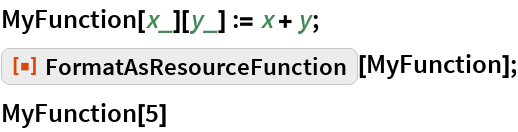Out=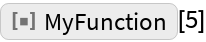Format using a different name:

 In:=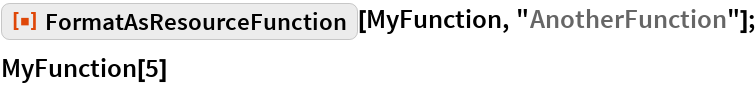Out=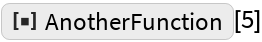Format with an interpretation as a specific resource:

 In:=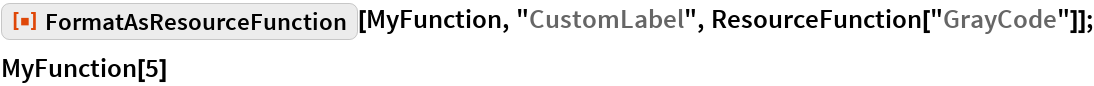Out=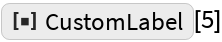Copy and paste the output above:

 In:=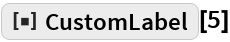Out=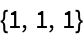This is the same as:

 In:=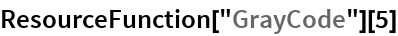Out=### Applications

Here is a function that has an unevaluated operator form:

 In:=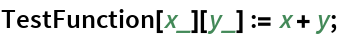In:=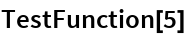Out=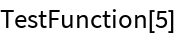In:=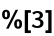Out=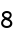Although it works correctly as a ResourceFunction, the operator form is represented by the internal symbol:

 In:=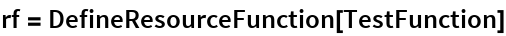Out=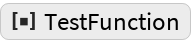In:=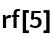Out=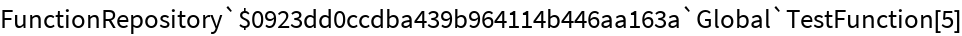In:=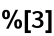Out=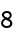Format the symbol so it will appear nicely in output:

 In:=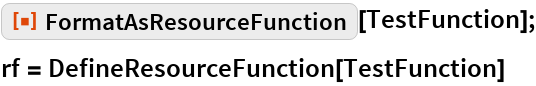Out=Now the operator formats as a ResourceFunction:

 In:=Out=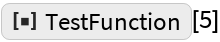In:=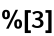Out=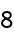Copy the output from above and paste into a Hold to see the interpretation:

 In:=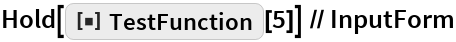Out=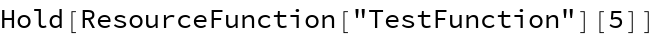### Possible Issues

Attempting to copy and paste the formatted symbol before the ResourceFunction exists will result in an error:

 In:=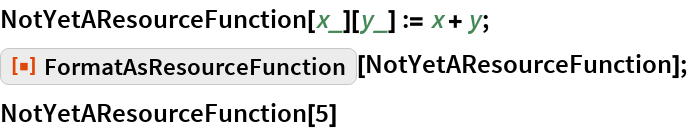Out=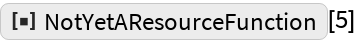Copy and paste the output from above:

 In:=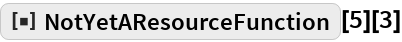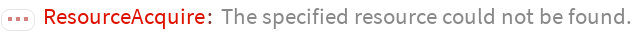Out=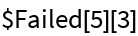Paste the same again, but this time define the ResourceFunction first and it will work:

 In:=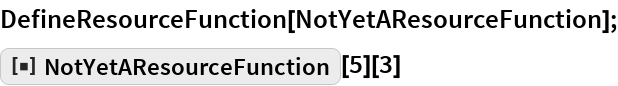Out=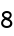## Requirements

Wolfram Language 11.3 (March 2018) or above